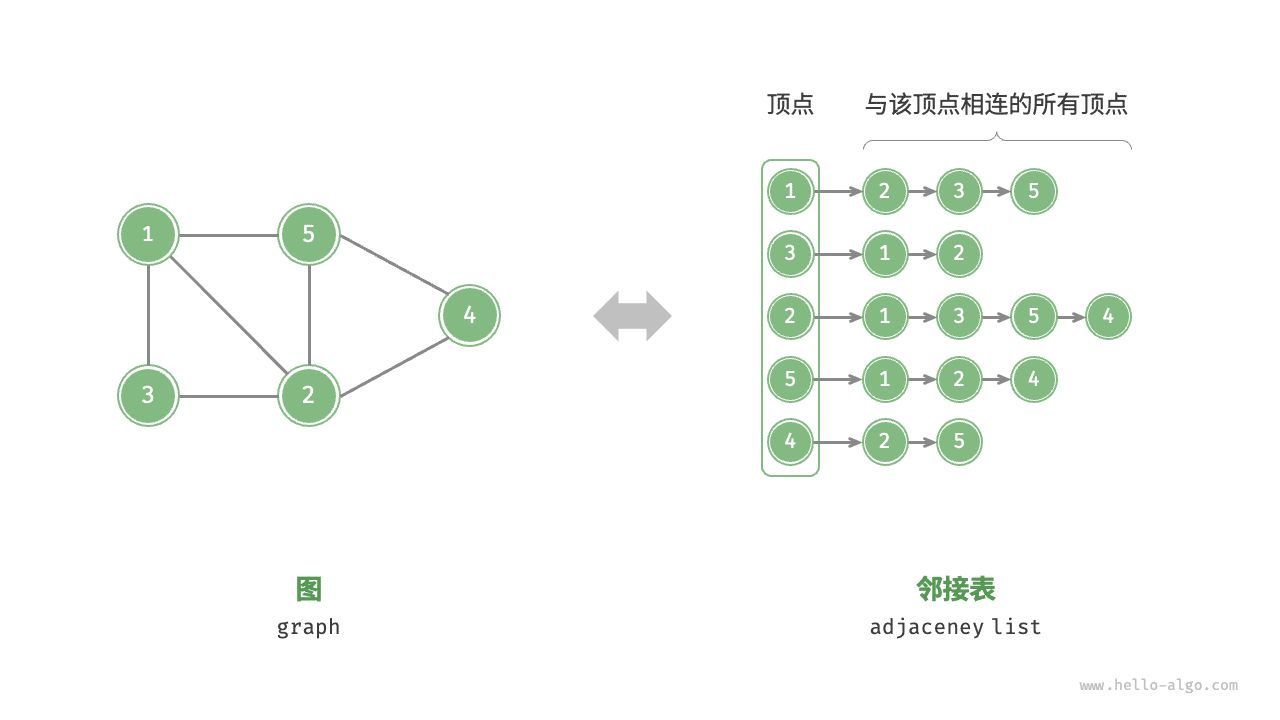# 9.1   图¶

「图 graph」是一种非线性数据结构，由「顶点 vertex」和「边 edge」组成。我们可以将图 $$G$$ 抽象地表示为一组顶点 $$V$$ 和一组边 $$E$$ 的集合。以下示例展示了一个包含 5 个顶点和 7 条边的图。

\begin{aligned} V & = \{ 1, 2, 3, 4, 5 \} \newline E & = \{ (1,2), (1,3), (1,5), (2,3), (2,4), (2,5), (4,5) \} \newline G & = \{ V, E \} \newline \end{aligned}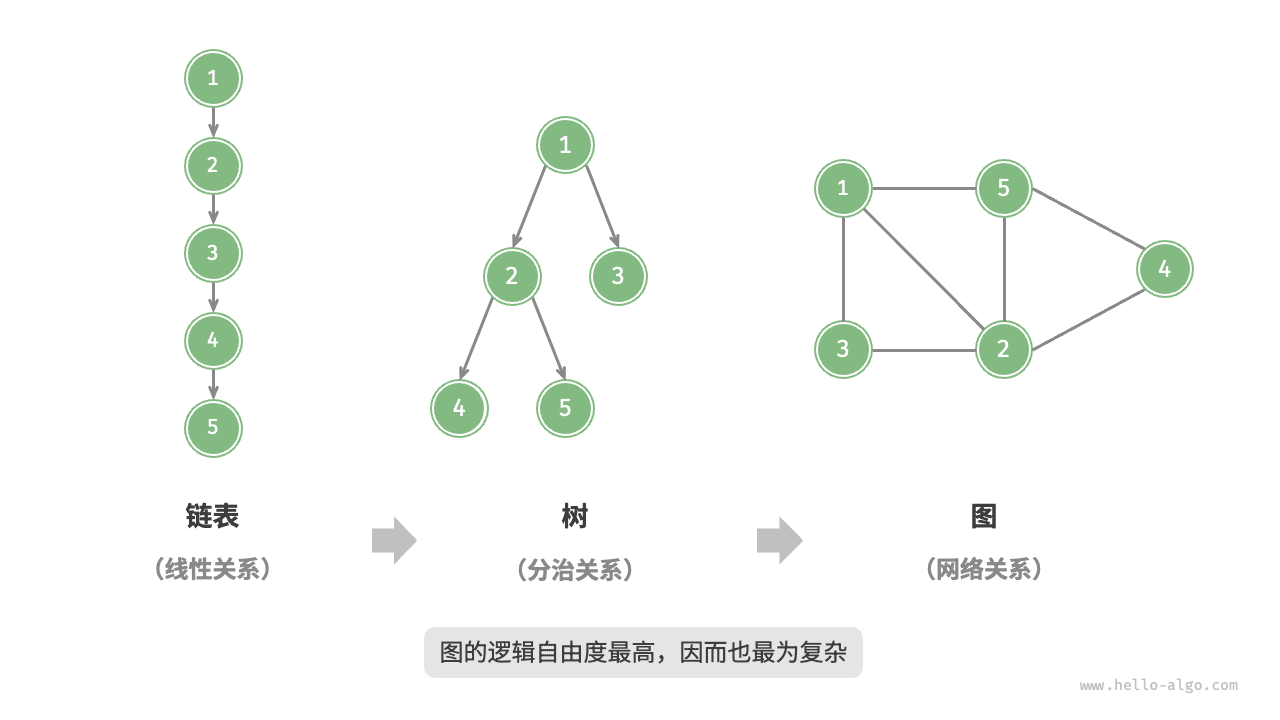## 9.1.1   图常见类型与术语¶

• 在无向图中，边表示两顶点之间的“双向”连接关系，例如微信或 QQ 中的“好友关系”。
• 在有向图中，边具有方向性，即 $$A \rightarrow B$$$$A \leftarrow B$$ 两个方向的边是相互独立的，例如微博或抖音上的“关注”与“被关注”关系。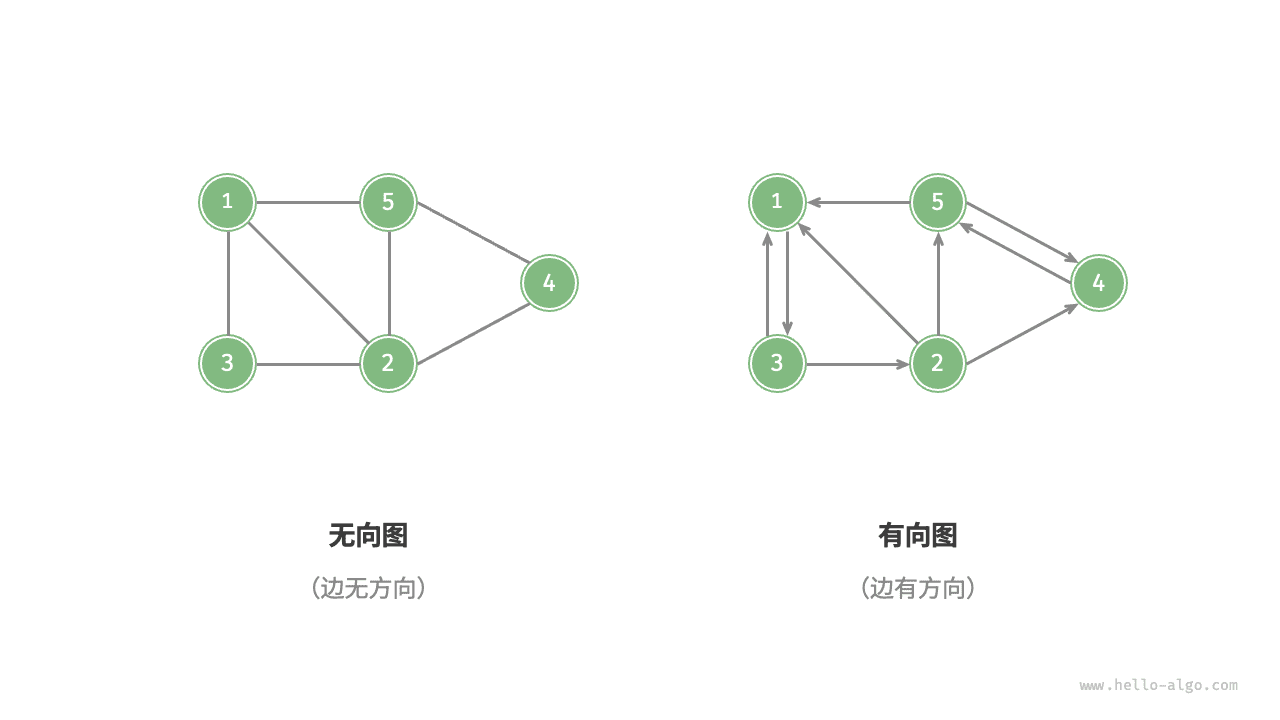• 对于连通图，从某个顶点出发，可以到达其余任意顶点。
• 对于非连通图，从某个顶点出发，至少有一个顶点无法到达。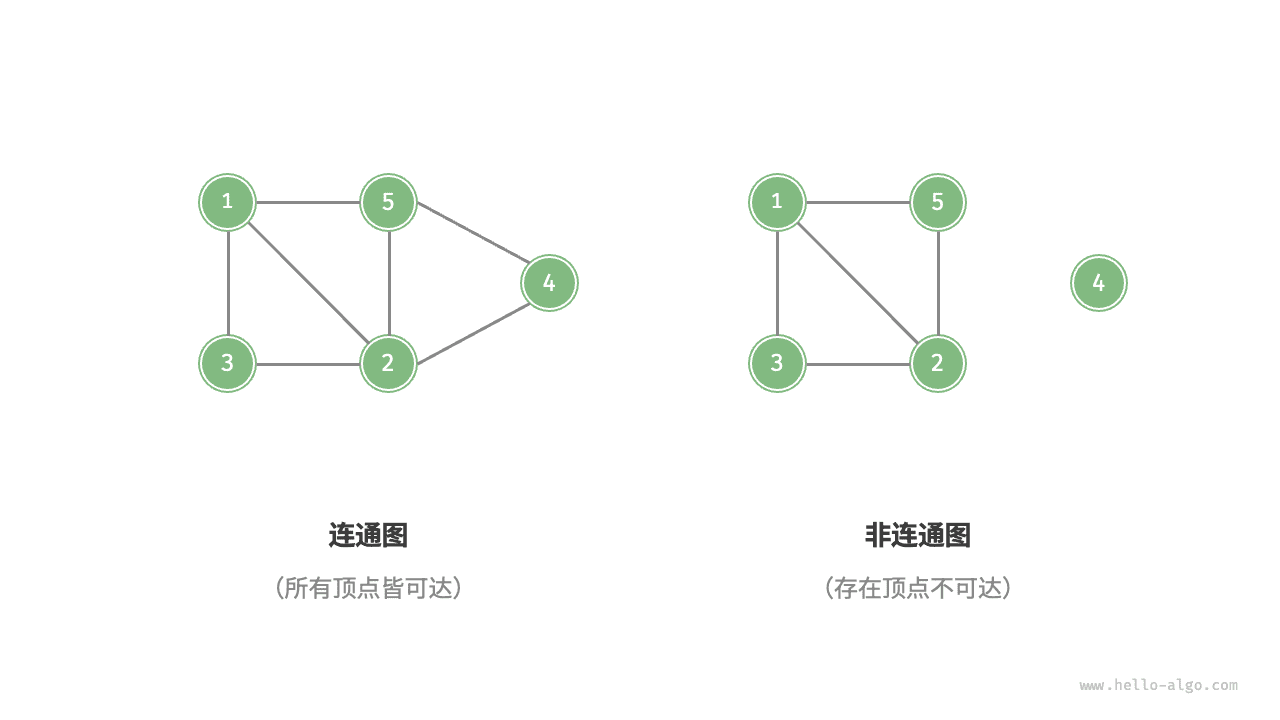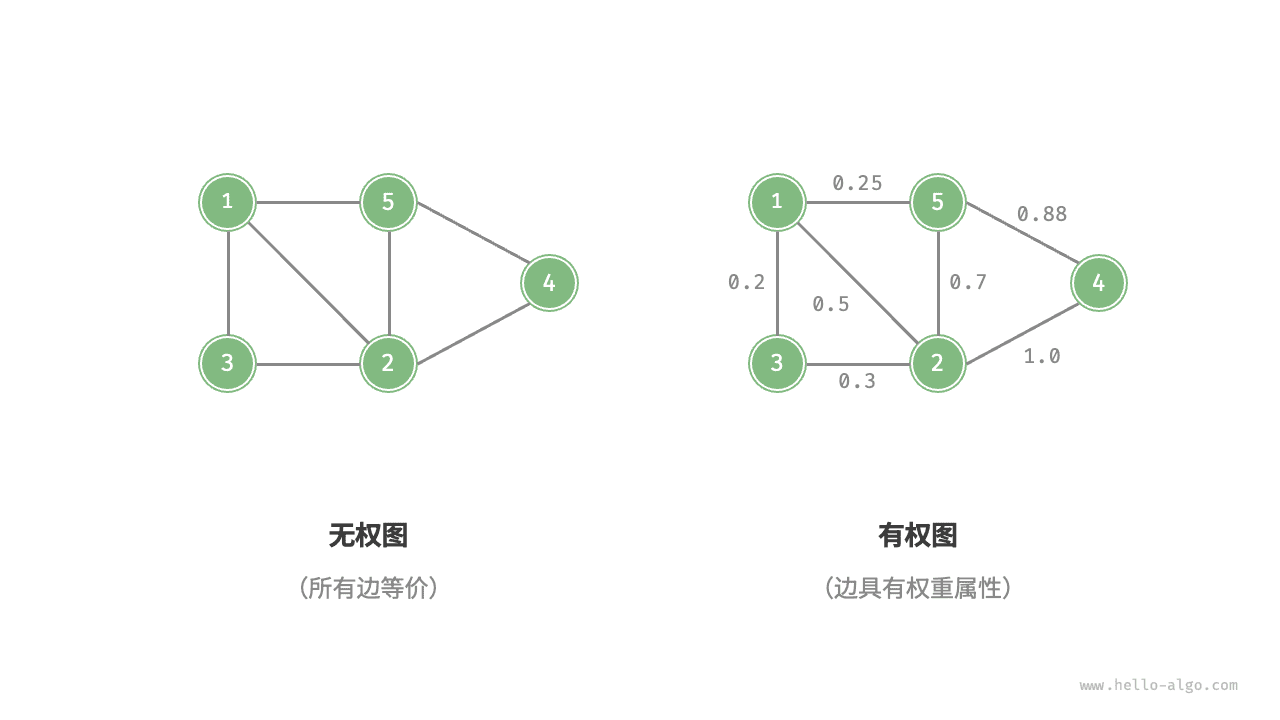• 「邻接 adjacency」：当两顶点之间存在边相连时，称这两顶点“邻接”。在图 9-4 中，顶点 1 的邻接顶点为顶点 2、3、5。
• 「路径 path」：从顶点 A 到顶点 B 经过的边构成的序列被称为从 A 到 B 的“路径”。在图 9-4 中，边序列 1-5-2-4 是顶点 1 到顶点 4 的一条路径。
• 「度 degree」：一个顶点拥有的边数。对于有向图，「入度 in-degree」表示有多少条边指向该顶点，「出度 out-degree」表示有多少条边从该顶点指出。

## 9.1.2   图的表示¶

### 1.   邻接矩阵¶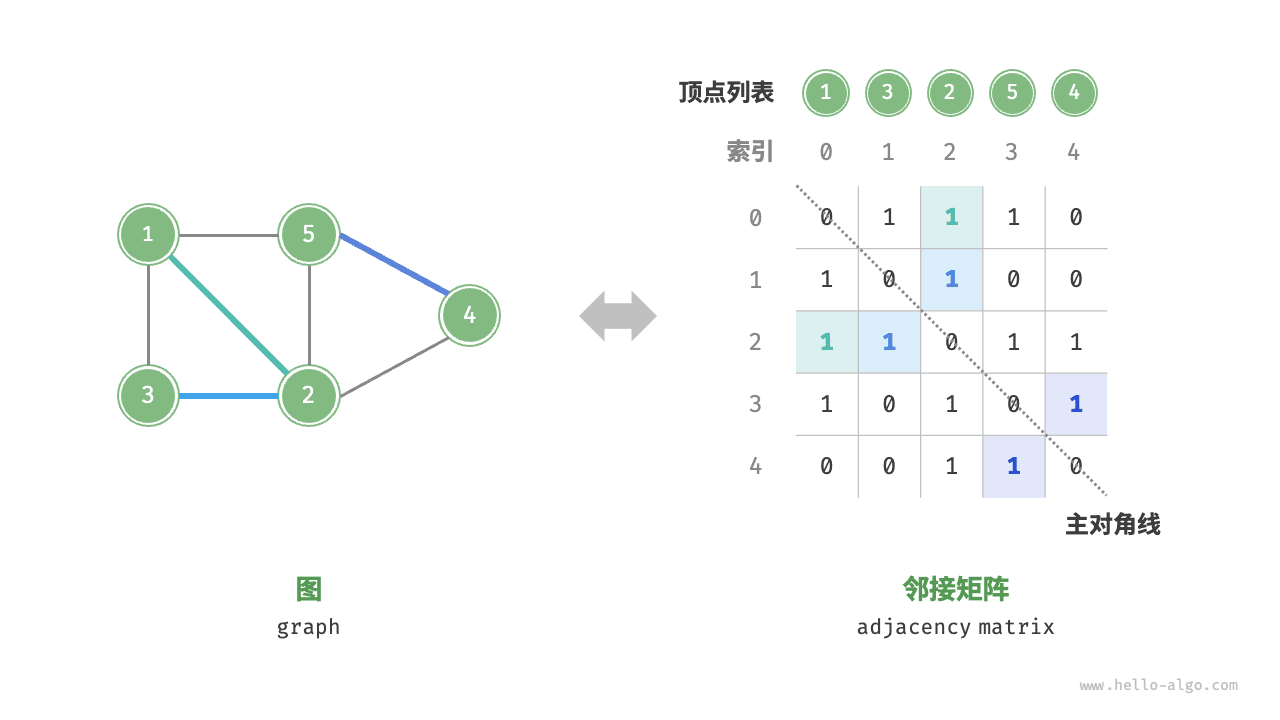• 顶点不能与自身相连，因此邻接矩阵主对角线元素没有意义。
• 对于无向图，两个方向的边等价，此时邻接矩阵关于主对角线对称。
• 将邻接矩阵的元素从 $$1$$$$0$$ 替换为权重，则可表示有权图。

### 2.   邻接表¶

「邻接表 adjacency list」使用 $$n$$ 个链表来表示图，链表节点表示顶点。第 $$i$$ 个链表对应顶点 $$i$$ ，其中存储了该顶点的所有邻接顶点（与该顶点相连的顶点）。图 9-6 展示了一个使用邻接表存储的图的示例。